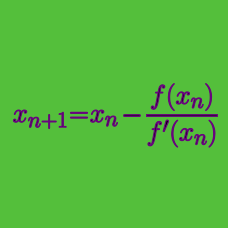Calculus

# Root Approximation: Level 3 Challenges

$\large \color{#D61F06}{3}x^{5} \color{#3D99F6}{=} \color{#D61F06}{1}x^{4} + \color{#D61F06}{4}x^{3} + \color{#D61F06}{1}x^{2} + \color{#D61F06}{5}x + \color{#D61F06}{9}$

The above polynomial has exactly one real root $\alpha$ . Find $\lfloor 1000 \times \alpha \rfloor$.

Notation: $\lfloor x \rfloor$ denotes the greatest integer smaller than or equal to $x$. This is known as the greatest integer function.

Consider this equation

$2^x=x^2$

There are 2 trivial solutions, namely $x=2$ and $x=4$

However, the equation is known to also have a negative solution. Find the negative solution.

Give your answer to 3 decimal places.

Find the value of $a$ to 3 significant figures, so that $x$ and $y$ are distinct real solutions to

$x+y=100\pi, \\ x^y = y^x = a.$

$\large x^y = y^x = 43$

If $x$ and $y$ are distinct positive real numbers satisfying the equation above, find $\lfloor 100(x+y) \rfloor$.

You are allowed to use computer assistance.

You might refer to Soumava's algorithm.

$f(x) = 9x^8 + 7x^6 + 5x^4 + 3x^2 - 1 = 0$

Which option is closest to the largest real root of the equation above?

×